# Forestry: Maximising the Area of a Duck Enclosure

We are planning to study feeding and growth in mallard ducks during nesting. We want to build a rectangular enclosure for the ducks so they will be safe from predators and can be studied. Also, we want the area of the enclosure to be as large as possible.

One side of the enclosure is along the straight edge of a lake. We have 120m of fencing available and need double fencing one metre apart on the three "land" sides of the enclosure to keep predators away. On the "water" side, we need only a single fence (see diagram below - fencing is in blue).

ENCLOSURE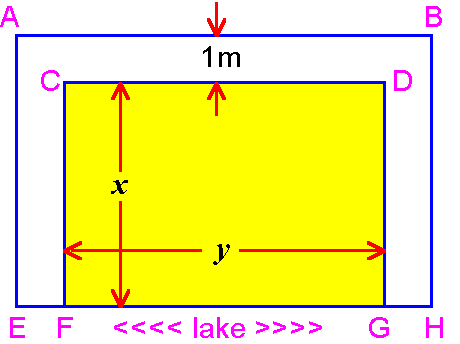## QUESTION:

To make the area of the enclosure as large as possible,

1. What must the dimensions x and y be?
2. What lengths are the seven straight sections of fence: AB, CD, EH, AE, CF, DG and BH?
3. What is the area of the enclosure?

## SOLUTION:

### Step 1:

Compute the lengths of fence used on each side in terms of the unknowns x and y.

1. Water side (EH): y+2 metres
2. Each side adjacent to the water side:
Inside fence (CF and DG): x metres
Outside fence (AE and BH): x+1 metres
3. Side opposite the water side:
Inside fence (CD): y metres
Outside fence (AB): y+2 metres

The total length of fencing is 120 metres, hence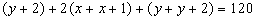or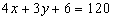(equation 1)

### Step 2:

Compute the area of the inside rectangle in terms of x and y.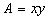(equation 2)

Now solve equation (1) for y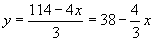(equation 3)

Substitute for y in equation (2). Then,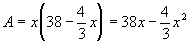### Step 3:

Find the extreme value(s) for area, A.

A will reach an extreme value (maximum or minimum) when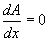Computing the derivative and setting it to zero,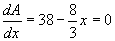(equation 4)

Solving for x,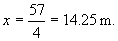Substituting into equation (3),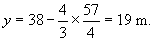### Step 4:

Verify that A has a maximum at x = 14.25 and not a minimum.

To do this, compute the second derivative,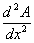and evaluate at x = 14.25. If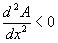it is a maximum; if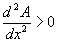it is a minimum. From (4),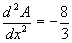for all x, hence we have a maximum.

For the enclosure to have maximum area,

1. The dimensions of the inside will be 14.25m by 19m.
2. The seven straight sections of fencing will have lengths:

AB and EH, 21m

AE and BH, 15.25m

CD, 19m

CF and DG 14.25m.

3. The area will be 270.75m2.Written by G. John Smith, September 3, 1998 - 10:24:07 PM